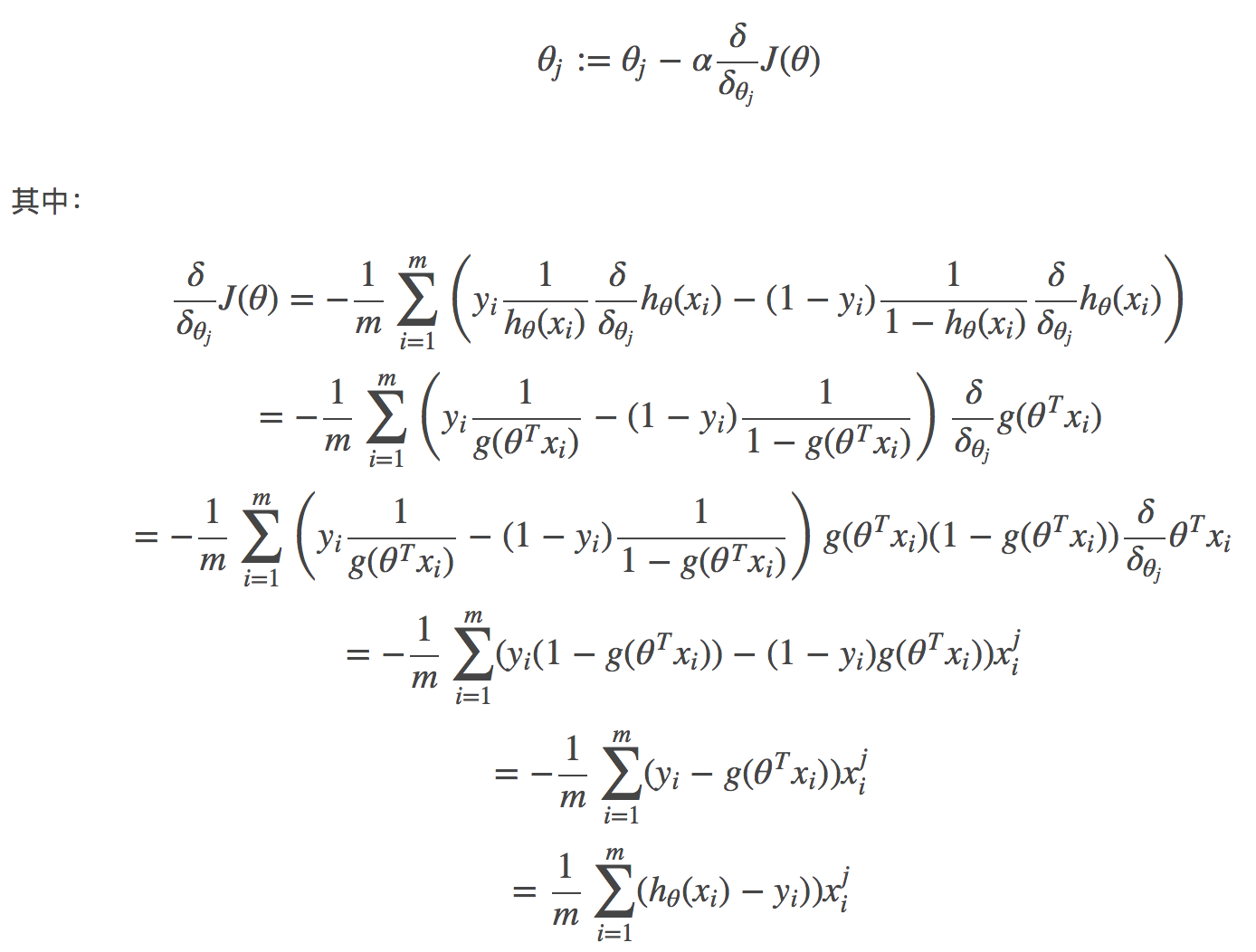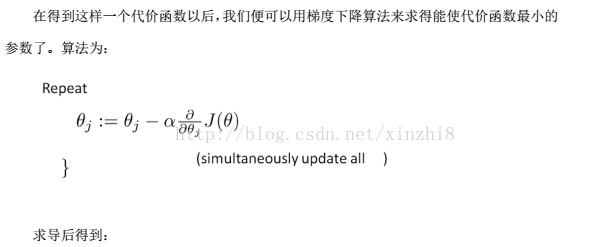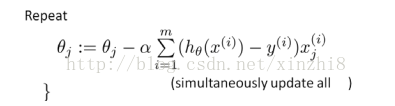# logistic回归损失函数与梯度下降

${h}_{\theta }\left(x\right)=g\left({\theta }^{\mathrm{T}}x\right)=\frac{1}{1+{e}^{-{\theta }^{\mathrm{T}}x}}=g\left({\theta }^{\mathrm{T}}x+b\right)$

${h}_{\theta }\left(x\right)=g\left({\theta }^{T}x\right)\ge 0.5$$h_θ(x)=g(θ^Tx)≥0.5$, 即${\theta }^{T}x\ge 0$$θ^Tx≥0$, 此时意味着预估$y=1$$y=1$;
${h}_{\theta }\left(x\right)=g\left({\theta }^{T}x\right)<0.5$$h_θ(x)=g(θ^Tx)<0.5$, 即${\theta }^{T}x<0$$θ^Tx<0$, 此时意味着预估$y=0$$y=0$.

$\left\{\begin{array}{l}P\left(y=1|x;\theta \right)={h}_{\theta }\left(x\right)\\ P\left(y=0|x;\theta \right)=1-{h}_{\theta }\left(x\right)\end{array}$

# 损失函数与梯度下降

1.损失函数(Loss function)是定义在单个训练样本上的，也就是就算一个样本的误差，比如我们想要分类，就是预测的类别和实际类别的区别，是一个样本的哦，用L表示
2.代价函数(Cost function)是定义在整个训练集上面的，也就是所有样本的误差的总和的平均，也就是损失函数的总和的平均，有没有这个平均其实不会影响最后的参数的求解结果。

1. 0-1损失函数（0-1 loss function）
2. 平方损失函数（quadratic loss function)
3. 绝对值损失函数(absolute loss function)
4. 对数损失函数（logarithmic loss function) 或对数似然损失函数(log-likehood loss function)

### 构造代价函数求出参数的值

#### 构造代价函数

$cost\left({h}_{\theta }\left(x\right),y\right)=\left\{\begin{array}{ll}-log\left({h}_{\theta }\left(x\right)\right)& \text{if y=1}\\ -log\left(1-{h}_{\theta }\left(x\right)\right)& \text{if y=0}\end{array}$

$cost\left({h}_{\theta }\left(x\right),y\right)=-{y}_{i}log\left({h}_{\theta }\left(x\right)\right)-\left(1-{y}_{i}\right)log\left(1-{h}_{\theta }\left(x\right)\right)$

$cost\left({h}_{\theta }\left(x\right),y\right)=\sum _{i=1}^{m}-{y}_{i}log\left({h}_{\theta }\left(x\right)\right)-\left(1-{y}_{i}\right)log\left(1-{h}_{\theta }\left(x\right)\right)$

$\left\{\begin{array}{l}P\left(y=1|x;\theta \right)={h}_{\theta }\left(x\right)\\ P\left(y=0|x;\theta \right)=1-{h}_{\theta }\left(x\right)\end{array}$

$P\left({y}_{i}|{x}_{i}\right)=\left\{\begin{array}{lll}{h}_{\theta }\left({x}_{i}\right)& & {y}_{i}=1\\ 1-{h}_{\theta }\left({x}_{i}\right)& & {y}_{i}=0\end{array}$

$P\left({y}_{i}|{x}_{i}\right)=\left({h}_{\theta }\left({x}_{i}\right){\right)}^{{y}_{i}}\left(1-{h}_{\theta }\left({x}_{i}\right){\right)}^{1-{y}_{i}}$

$L=\prod _{i=1}^{m}P\left({y}_{i}|{x}_{i}\right)=\prod _{i=1}^{m}\left({h}_{\theta }\left({x}_{i}\right){\right)}^{{y}_{i}}\left(1-{h}_{\theta }\left({x}_{i}\right){\right)}^{1-{y}_{i}}$

$l=\sum _{i=1}^{m}\left({y}_{i}log\left({h}_{\theta }\left({x}_{i}\right)\right)+\left(1-{y}_{i}\right)log\left(1-{h}_{\theta }\left({x}_{i}\right)\right)\right)$

#### 求出参数的值

https://blog.csdn.net/lookqlp/article/details/51161640

1. 寻找预测函数（h函数，hypothesis）
2. 构造损失函数（J函数）
3. 使损失函数最小，获得回归系数θ

1. 梯度下降
2. 牛顿迭代算法
3. 拟牛顿迭代算法（BFGS算法和L-BFGS算法）

$\begin{array}{rl}J\left(\theta \right)& =-\frac{1}{m}l\left(\theta \right)\\ & =-\frac{1}{m}logL\left(\theta \right)\\ & =-\frac{1}{m}\sum _{i=1}^{m}\left({y}_{i}log\left({h}_{\theta }\left(x\right)\right)+\left(1-{y}_{i}\right)log\left(1-{h}_{\theta }\left(x\right)\right)\right)\end{array}$${\theta }_{j}:={\theta }_{j}-\alpha \frac{1}{m}\sum _{i=1}^{m}\left({h}_{\theta }\left({x}_{i}\right)-{y}_{i}\right){x}_{i}^{j}$